Most Affordable JEE | NEET | 8,9,10 Preparation by Kota's Top IITian Doctor Faculties

# Chemical Bonding - JEE Advanced Previous Year Questions with Solutions`
JEE Advanced Previous Year Questions of Chemistry with Solutions are available at eSaral. Practicing JEE Advanced Previous Year Papers Questions of Chemistry will help the JEE aspirants in realizing the question pattern as well as help in analyzing weak & strong areas. Simulator   Previous Years IIT/JEE Advance Questions
Q. Match each of the diatomic molecules in Column I with its property / properties in Column II.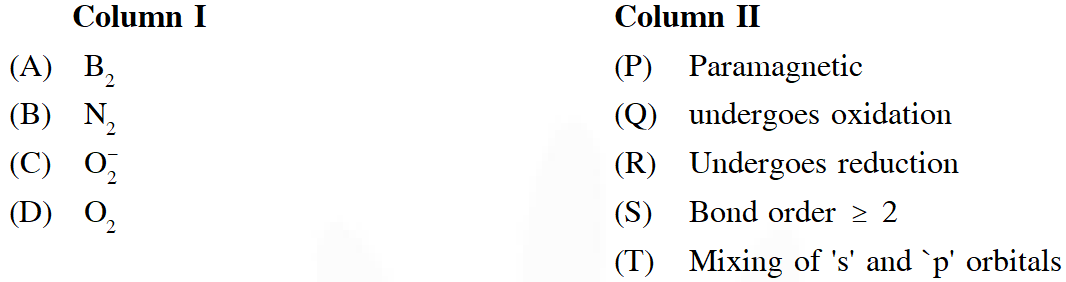[JEE 2009]
Ans. $A \rightarrow P, Q, R, S$ $B \rightarrow Q, R, S, T$ $C \rightarrow P, Q, R$ $D \rightarrow P, Q, R, S$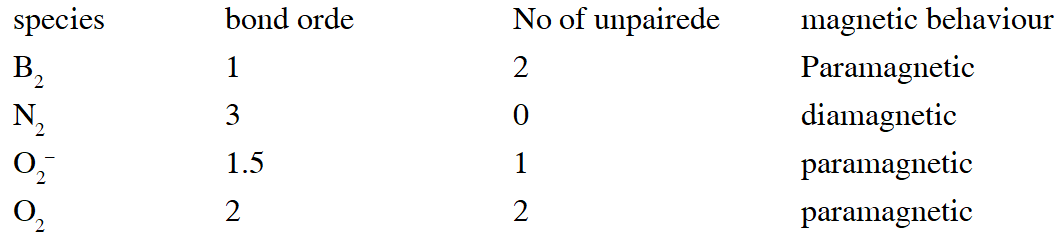Q. The nitrogen oxide(s) that contain(s) N–N bond(s) is (are) (A) $\mathrm{N}_{2} \mathrm{O}$ (B) $\mathrm{N}_{2} \mathrm{O}_{3}$ (C) $\mathrm{N}_{2} \mathrm{O}_{4}$ (D) $\mathrm{N}_{2} \mathrm{O}_{5}$ [JEE 2009]
Ans. (A,B,C)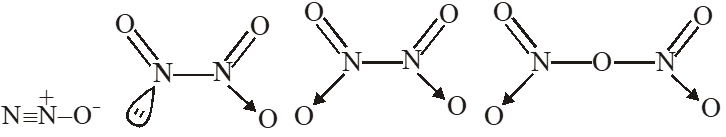Q. In the reaction $2 \mathrm{X}+\mathrm{B}_{2} \mathrm{H}_{6} \longrightarrow\left[\mathrm{BH}_{2}(\mathrm{X})_{2}\right]^{+}\left[\mathrm{BH}_{4}\right]^{-}$ the amine(s) X is (are) (A) $\mathrm{NH}_{3}$ (B) $\mathrm{CH}_{3} \mathrm{NH}_{2}$ (C) $\left(\mathrm{CH}_{3}\right)_{2} \mathrm{NH}$ $(\mathrm{D})\left(\mathrm{CH}_{3}\right)_{3} \mathrm{N}$ [JEE 2009]
Ans. (B,C)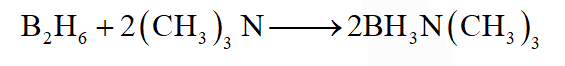Q. The number of water molecule(s) directly bonded to the metal centre in $\mathrm{CuSO}_{4} \cdot 5 \mathrm{H}_{2} \mathrm{O}$ is [JEE 2009]
Ans. (4)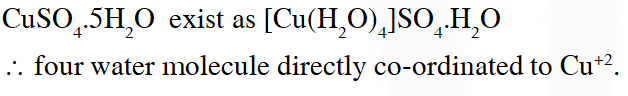Q. The species having pyramidal shape is (A) $\mathrm{SO}_{3}$ (B) $\operatorname{BrF}_{3}$ (C) $\mathrm{SiO}_{3}^{2-}$ (D) $\mathrm{OSF}_{2}$ [JEE 2010]
Ans. (D)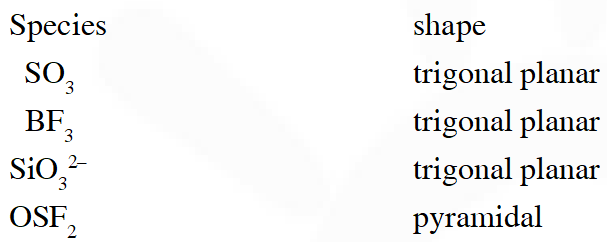Q. Assuming that Hund's rule is violated, the bond order and magnetic nature of the diatomic molecule $\mathrm{B}_{2}$ is (A) 1 and diamagnetic (B) 0 and diamagnetic (C) 1 and paramagnetic (D) 0 and paramagnetic [JEE 2010]
Ans. (A) If Hund's rule is violated in Boron then unpaired electron of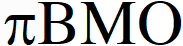gets paired up and boron becomes diamagnetic.
Q. Based on VSEPR theory, the number of 90 degree F–Br–F angles in $\mathrm{BrF}_{5}$ is [JEE 2010]
Ans. 0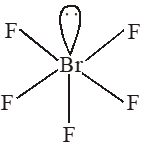According to VSEPR theory all bond angle are $<90 \%$
Q. The value of n in the molecular formula $\mathrm{Be}_{\mathrm{n}} \mathrm{Al}_{2} \mathrm{Si}_{6} \mathrm{O}_{18}$ is [JEE 2010]
Ans. 3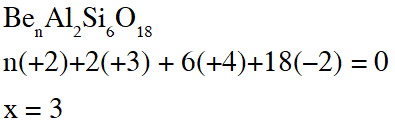Q. The total number of diprotic acids among the following is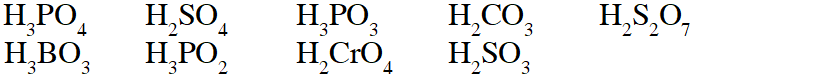[JEE 2010]
Ans. 6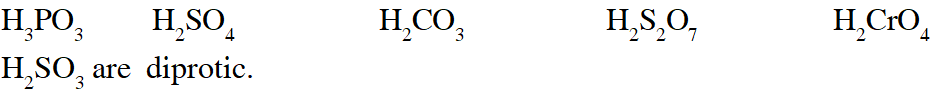Q. Among the following, the number of elements showing only one non-zero oxidation state is O, Cl, F, N, P, Sn, Tl, Na, Ti [JEE 2010]
Ans. 2 Na & F
Q. The difference in the oxidation numbers of the two types of sulphur atoms in $\mathrm{Na}_{2} \mathrm{S}_{4} \mathrm{O}_{6}$ is. [JEE 2011]
Ans. 5 $\mathrm{Na}_{2} \mathrm{S}_{4} \mathrm{O}_{6}$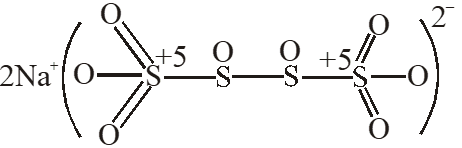Q. Which ordering of compounds is according to the decreasing order of the oxidation state of nitrogen- (A) $\mathrm{HNO}_{3}, \mathrm{NO}, \mathrm{NH}_{4} \mathrm{Cl}, \mathrm{N}_{2}$ (B) $\mathrm{HNO}_{3}, \mathrm{NO}, \mathrm{N}_{2}, \mathrm{NH}_{4} \mathrm{Cl}$ (C) $\mathrm{HNO}_{3}, \mathrm{NH}_{4} \mathrm{Cl}, \mathrm{NO}, \mathrm{N}_{2}$ (D) $\mathrm{NO}, \mathrm{HNO}_{3}, \mathrm{NH}_{4} \mathrm{Cl}, \mathrm{N}_{2}$ [JEE 2012]
Ans. (B)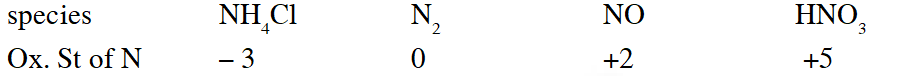Q. In allene $\left(\mathrm{C}_{3} \mathrm{H}_{4}\right)$, the type(s) of hybridisation of the carbon atoms is (are) (A) sp and $\mathrm{sp}^{3}$ (B) sp and $\mathrm{sp}^{2}$ (C) only $\mathrm{sp}^{2}$ (D) $\mathrm{sp}^{2}$ and $\mathrm{sp}^{3}$ [JEE 2012]
Ans. (B)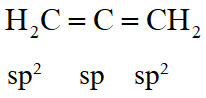Q. The shape of $\mathrm{XeO}_{2} \mathrm{F}_{2}$ molecule is : (A) Trigonal bipyramidal (B) Square planar (C) tetrahedral (D) see-saw [JEE 2012]
Ans. (D)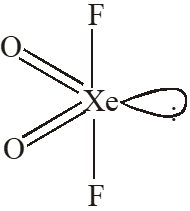$\mathrm{sp}^{3} \mathrm{d} \quad$ See-Saw shape
Q. The total number of lone-pairs of electrons in melamine is [JEE Adv. 2013]
Ans. 6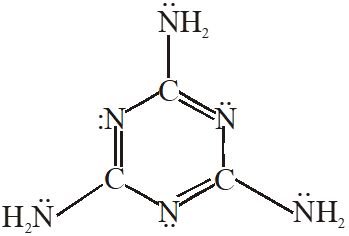Q. Assuming 2s-2p mixing is NOT operative, the paramagnetic species among the following is (A) $\mathrm{Be}_{2}$ (B) $\mathrm{B}_{2}$ (C) $\mathrm{C}_{2}$ (D) $\mathrm{N}_{2}$ [JEE Adv. 2014]
Ans. (C) If 2s–2p is not operative than $\mathrm{C}_{2}$ becomes paramagnetic.
Q. Match the orbital overlap figures shown in List-I with the description given in List-II and select the correct answer using the code given below the lists.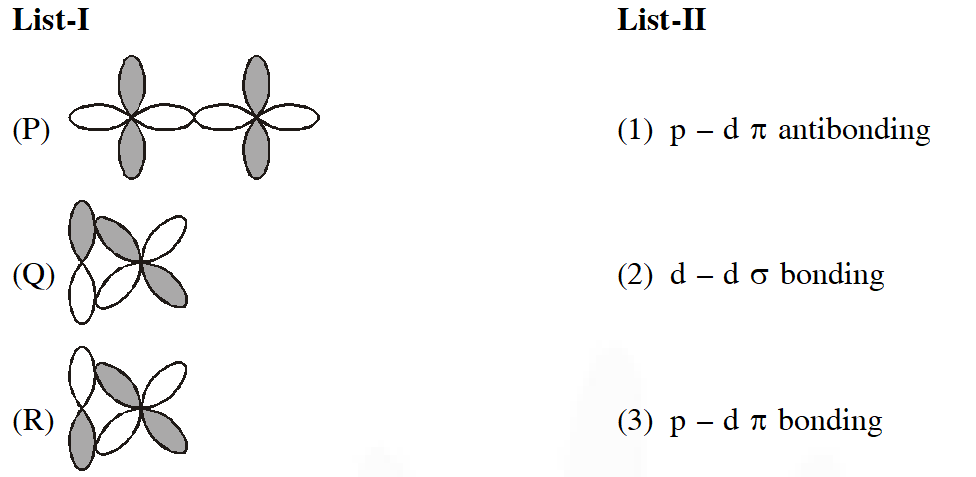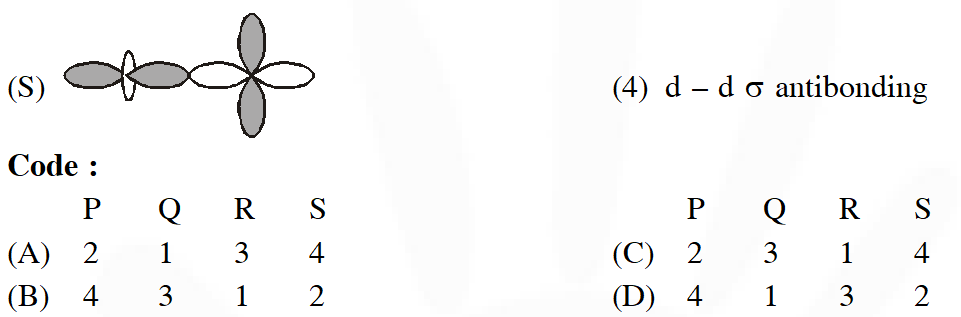[JEE Adv. 2014]
Ans. (C)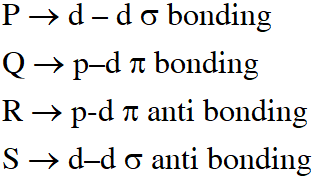Q. The compound(s) with TWO lone pairs of electrons on the central atom is(are) – (A) $\mathrm{BrF}_{5}$ (B) $\mathrm{ClF}_{3}$ (C) $\mathrm{XeF}_{4}$ (D) SF $_{4}$ [JEE - ADV. 2016]
Ans. (B,C)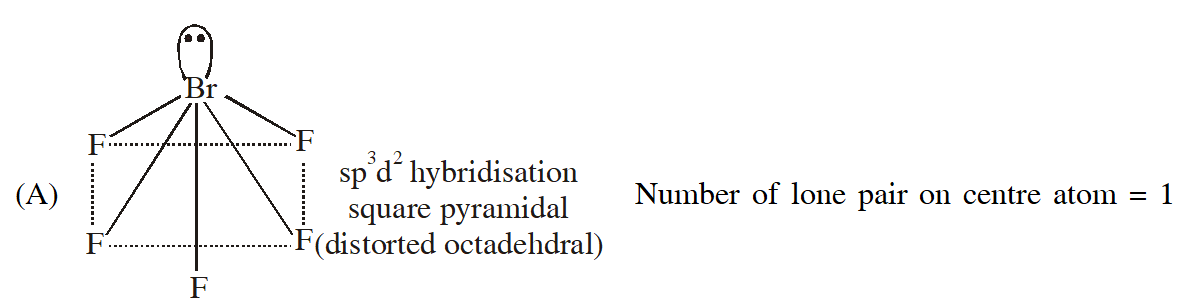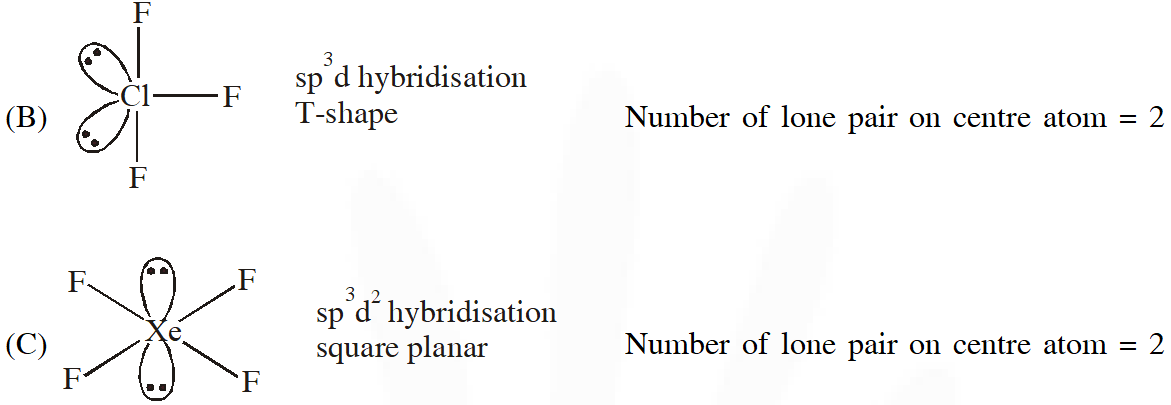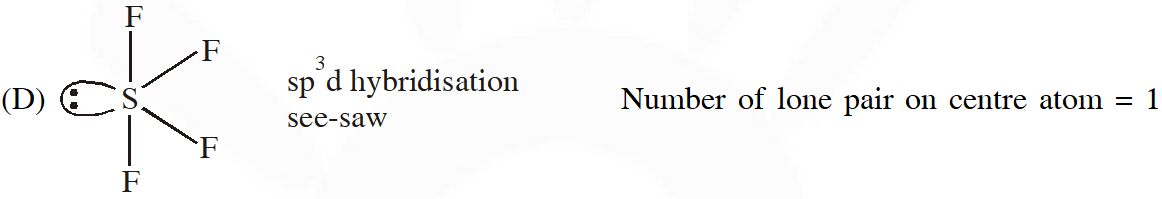Hence Ans. (B,C)
Q. The crystalline form of borax has (A)Tetranuclear$\left[\mathrm{B}_{4}\mathrm{O}_{5}(\mathrm{OH})_{4}\right]^2-}$ unit (B) All boron atoms in the same plane (C) Equal number of $\operatorname{sp}^{2}$ and $\mathrm{sp}^{3}$ hybridized boron atoms (D) One terminal hydroxide per boron atom [JEE - ADV. 2016]
Ans. (A,C,D)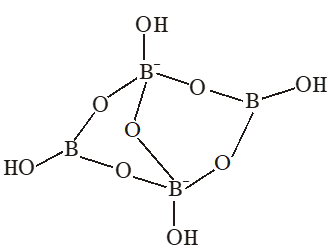(A)Having $\left[\mathrm{B}_{4} \mathrm{O}_{5}(\mathrm{OH})_{4}\right]^{2-}$ tetranuclear (boron) unit (B) All boron atoms not in same plane (C) Two boron are $\mathrm{Sp}^{2}$ hybridised and two boron are sp3 hybridised (D) One terminal hydroxide per boron atom is present.
Q. According to Molecular Orbital Theory, (A) $\mathrm{C}_{2}^{2-}$ is expected to be diamagnetic (B) $\mathrm{O}_{2}^{2+}$ is expected to have a longer bond length than O2 (C) $\mathrm{N}_{2}^{+}$ and $\mathrm{N}_{2}$– have the same bond order (D) $\mathrm{He}_{2}^{+}$ has the same energy as two isolated He atoms [JEE - ADV. 2016]
Ans. (A,C)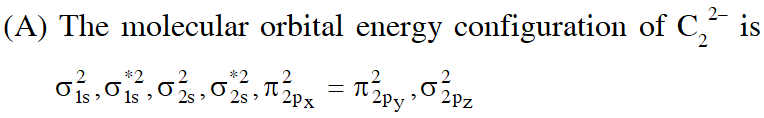In the MO of $\mathrm{C}_{2}^{2-}$ there is no unpaired electron hence it is diamagnatic (B) Bond order of $\mathrm{O}_{2}^{2+}$ is 3 and $\mathrm{O}_{2}$ is 2 therefore bond length of $\mathrm{O}_{2}$ is greater than $\mathrm{O}_{2}^{2+}$ (C) The molecular orbital energy configuration of $\mathrm{N}_{2}^{+}$ is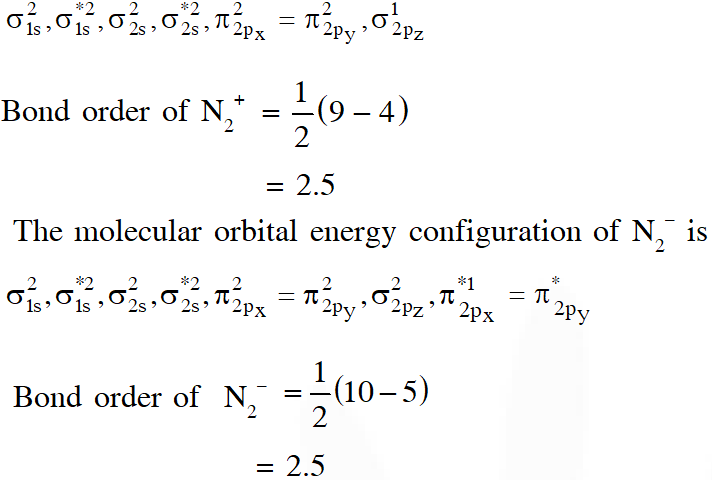(D) $\mathrm{He}_{2}^{+}$ has less energy as compare to two isolated He atoms
Q. The correct statements(s) about the oxoacids, $\mathrm{HClO}_{4}$ and HClO, is (are) – (A) $\mathrm{HClO}_{4}$ is more acidic than HClO because of the resonance stabilization of its anion (B) $\mathrm{HClO}_{4}$ is formed in the reaction between $\mathrm{Cl}_{2}$ and $\mathrm{H}_{2} \mathrm{O}$ (C) The central atom in Both $\mathrm{HClO}_{4}$ and HClO is $\mathrm{sp}^{3}$ hybridized (D) The conjugate base of $\mathrm{HClO}_{4}$ is weaker base than $\mathrm{H}_{2} \mathrm{O}$ [JEE - Adv. 2017]
Ans. (A,C,D)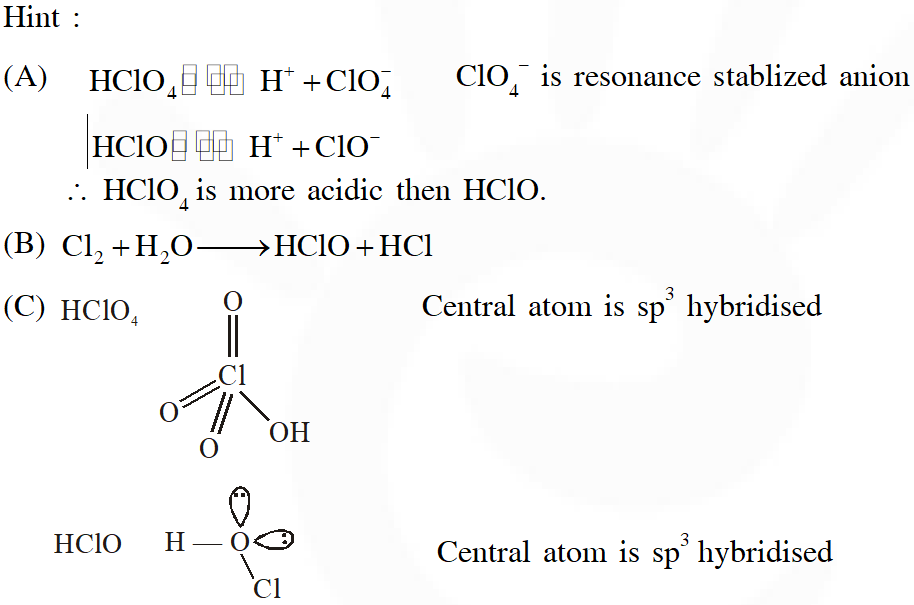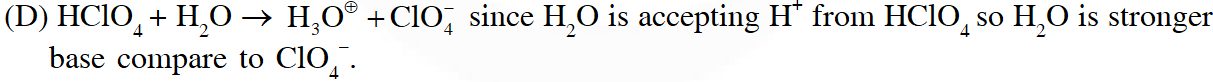Q. The colour of the $\mathrm{X}_{2}$ molecules of group 17 elements changes gradually from yellow to violet down the group. This is due to – A) the physical state of $\mathrm{x}_{2}$ at room temperature changes from gas to solid down the group (B) decrease in HOMO-LUMO gap down the group (C) decrease in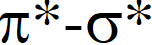down the group (D) decrease in ionization energy down the group [JEE - Adv. 2017]
Ans. (B,C)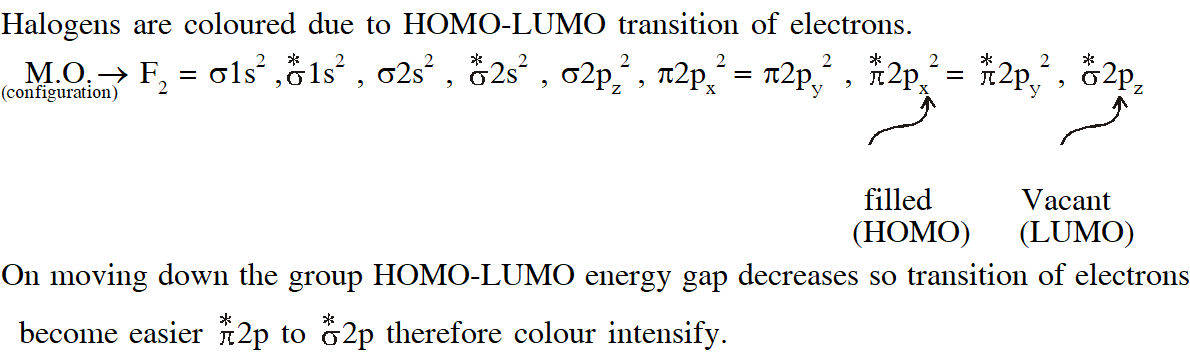Q.Among$\mathrm{H}_{2},\mathrm{He}_{2}^{+},\mathrm{Li}_{2},\mathrm{Be _{2},\mathrm{B}_{2},\mathrm{C}_{2}, \mathrm{N}_{2}, \mathrm{O}_{2}^{-}$ the number of diamagnetic species is - (Atomic number) : H = 1, He = 2, Li = 3, Be = 4, B = 5, C = 6, N = 7, O = 8 , f = 9) [JEE - Adv. 2017]
Ans. 5or6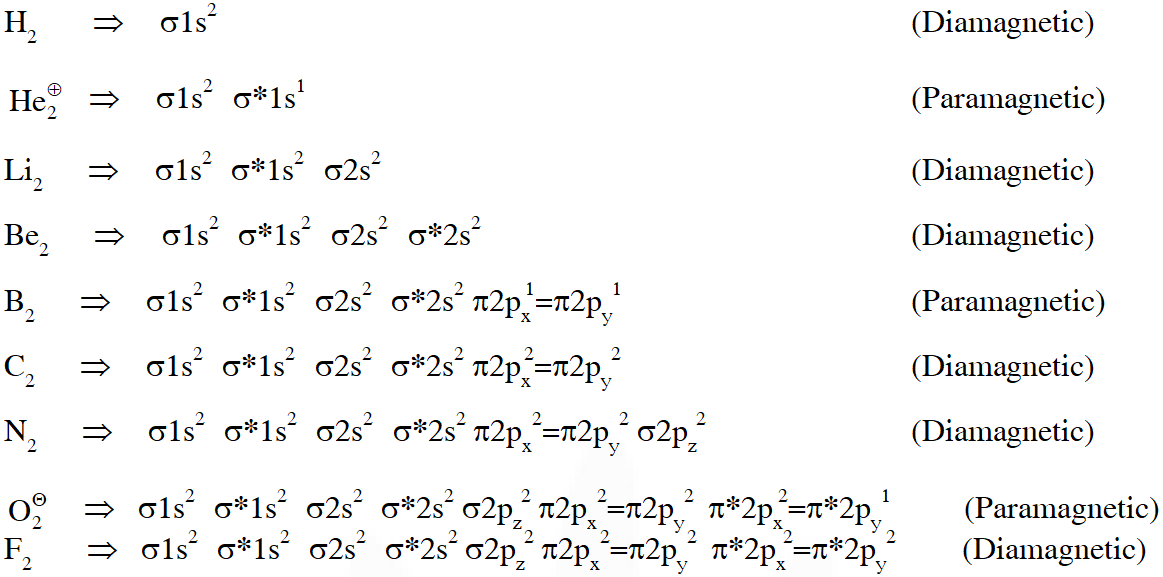If existence of $\mathrm{Be}_{2}$ is considered in atomic form or very weak bonded higher energetic species having zero bond order then it is diamagnetic , then answer will be 6. But if existence of molecular form of $\mathrm{Be}_{2}$ is not considered then magnetic property can't be predicted then answer will be 5.
Q. The sum of the number of lone pairs of electrons on each central atom in the following species is. $\left[\mathrm{TeBr}_{6}\right]^{2-},\left[\mathrm{BrF}_{2}\right]^{+}, \mathrm{SNF}_{3}$ and $\left[\mathrm{XeF}_{3}\right]^{-}$ [Atomic number : N = 7, F = 9, S = 16, Br = 35, Te = 52, Xe = 54] [JEE - Adv. 2017]
Ans. 6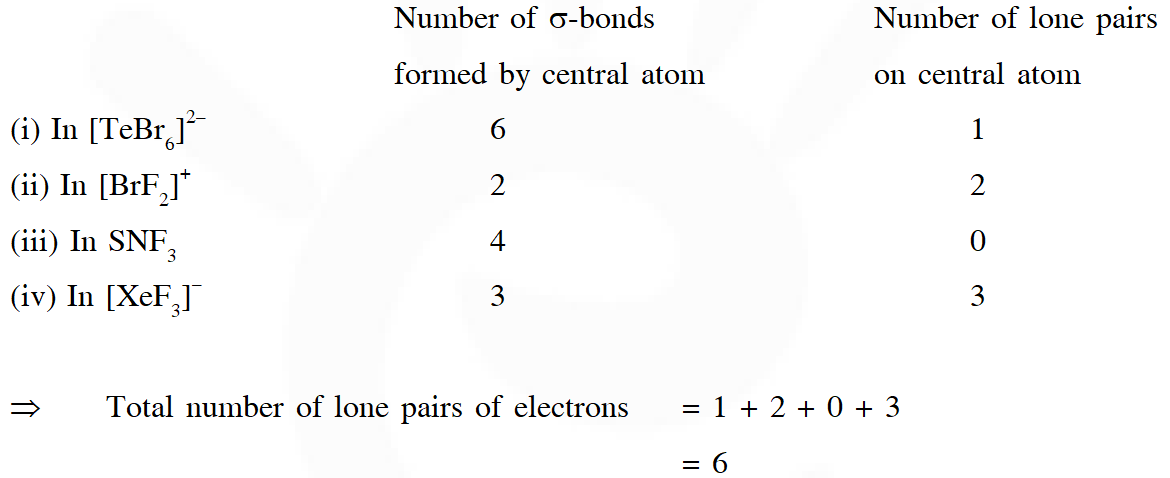Q. The order of the oxidation state of the phosphorus atom in $\mathrm{H}_{3} \mathrm{PO}_{2}, \mathrm{H}_{3} \mathrm{PO}_{4}, \mathrm{H}_{3} \mathrm{PO}_{3}$ and $\mathrm{H}_{4} \mathrm{P}_{2} \mathrm{O}_{6}$ is (A) $\mathrm{H}_{3} \mathrm{PO}_{4}>\mathrm{H}_{4} \mathrm{P}_{2} \mathrm{O}_{6}>\mathrm{H}_{3} \mathrm{PO}_{3}>\mathrm{H}_{3} \mathrm{PO}_{2}$ (B) $\mathrm{H}_{3} \mathrm{PO}_{3}>\mathrm{H}_{3} \mathrm{PO}_{2}>\mathrm{H}_{3} \mathrm{PO}_{4}>\mathrm{H}_{4} \mathrm{P}_{2} \mathrm{O}_{6}$ (C) $\mathrm{H}_{3} \mathrm{PO}_{2}>\mathrm{H}_{3} \mathrm{PO}_{3}>\mathrm{H}_{4} \mathrm{P}_{2} \mathrm{O}_{6}>\mathrm{H}_{3} \mathrm{PO}_{4}$ (D) $\mathrm{H}_{3} \mathrm{PO}_{4}>\mathrm{H}_{3} \mathrm{PO}_{2}>\mathrm{H}_{3} \mathrm{PO}_{3}>\mathrm{H}_{4} \mathrm{P}_{2} \mathrm{O}_{6}$ [JEE - Adv. 2017]
Ans. (A)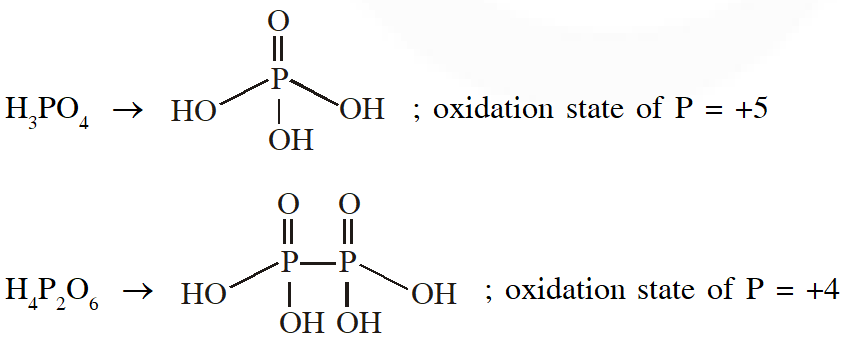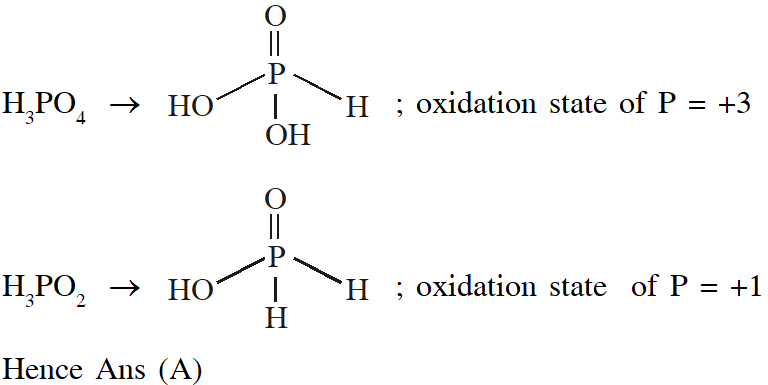Q. The option(s) with only amphoteric oxides is (are) (A) $\mathrm{Cr}_{2} \mathrm{O}_{3}, \mathrm{CrO}, \mathrm{SnO}, \mathrm{PbO}$ (B) $\mathrm{NO}, \mathrm{B}_{2} \mathrm{O}_{3}, \mathrm{PbO}, \mathrm{SnO}_{2}$ (C) $\mathrm{Cr}_{2} \mathrm{O}_{3}, \mathrm{BeO}, \mathrm{SnO}, \mathrm{SnO}_{2}$ (D) $\mathrm{ZnO}, \mathrm{Al}_{2} \mathrm{O}_{3}, \mathrm{PbO}, \mathrm{PbO}_{2}$ [JEE - Adv. 2017]
Ans. (A,C)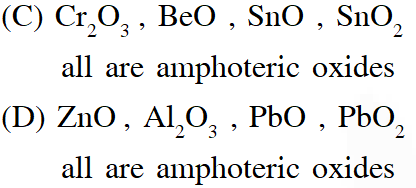Q. Among the following, the correct statement(s) is are (A) $\mathrm{Al}\left(\mathrm{CH}_{3}\right)_{3}$ has the three-centre two-electron bonds in its dimeric structure (B) $\mathrm{AlCl}_{3}$ has the three-centre two-electron bonds in its dimeric structure (C) BH3 has the three-centre two-electron bonds in its dimeric structure (D) The Lewis acidity of $\mathrm{BCl}_{3}$ is greater than that of $\mathrm{AlCl}_{3}$ [JEE - Adv. 2017]
Ans. (A,C,D) (A)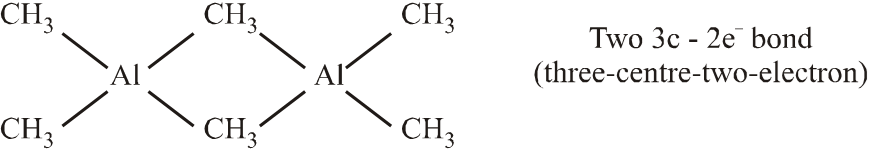(B)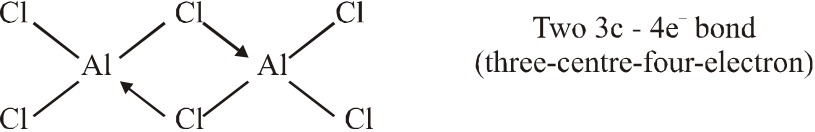(C)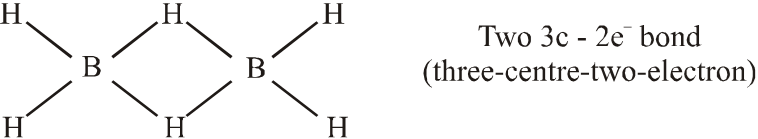(D) Lewis acidic strength decreases down the group. The decrease in acid strength occurs because as size increases, the attraction between the incoming electron pair and the nucleus weakens. Hence Lewis acidic strength of $\mathrm{BCl}_{3}$ is more than $\mathrm{AlCl}_{3}$.
Q. Based on the compounds of group 15 elements , the correct statement(s) is (are) (A) $\mathrm{Bi}_{2} \mathrm{O}_{5}$ is more basic than $\mathrm{N}_{2} \mathrm{O}_{5}$ (B) $\mathrm{NF}_{3}$ is more covalent than $\mathrm{BiF}_{3}$ (C) PH3 boils at lower temperature than $\mathrm{NH}_{3}$ (D) The N–N single bond is stronger than the P–P single bond [JEE - Adv. 2018]
Ans. (A,B,C)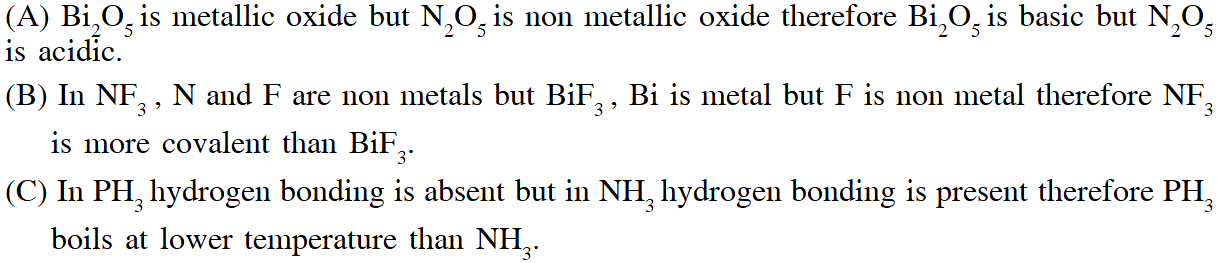(D) Due to small size in N–N single bond l.p. – l.p. repulsion is more than P–P single bond therefore N–N single bond is weaker than the P–P single bond.

tabasum ali .
March 11, 2023, 7:29 p.m.
thank you sir its very help full for me
Mamun
Aug. 16, 2022, 6:46 a.m.
Vgd
PRANAY RUPAVATH
Oct. 2, 2021, 2:02 p.m.
This is so helpful for a quick revision and helps to find a way to approach a question Thank you for this 💜
Sriharsha Rao
Sept. 12, 2021, 8:09 a.m.
thank you
Sriharsha Rao
Sept. 12, 2021, 8:09 a.m.
thanks
PAPPU BIHARI
Nov. 29, 2020, 12:39 p.m.
abey tumhare baap ka property hai ki copy option block karke rakhe ho. bake question chapterwise segregate karke achcha kiye ho. god bless you
Omnipresent
Oct. 15, 2020, 10:02 a.m.
Sounds great ,wishing to uptade even recent papers
Unknown
Oct. 4, 2020, 6:20 p.m.
Very Helpful, Much Appreciated, Thank You!
Ujjwal singh
Sept. 24, 2020, 9:41 a.m.
Thanks 😊
Jagdish
Sept. 21, 2020, 1:58 p.m.
thanks to you
ghyjgh
Sept. 18, 2020, 3:25 p.m.
nice
munna bhai
Sept. 6, 2020, 6:33 a.m.
THANKU SIR FOR THE ABOVE THEY ARE EASY SUMS